Home Practice
For learners and parents For teachers and schools
Textbooks
Full catalogue
Pricing SupportLog in

We think you are located in United States. Is this correct?

# 1.7 Percentages

## 1.7 Percentages (EMGZ)

Percentage
A number represented as a part of $$\text{100}$$.

### How to calculate a percentage of an amount (EMG32)

1. Write the percentage as a fraction with the denominator $$\text{100}$$, for example $$\text{20}\% = \frac{\text{20}}{\text{100}}$$. OR write the percentage as a decimal fraction, for example $$\text{20}\% = \text{0,2}$$.
2. Multiply this fraction / decimal fraction with the amount that is given.

Let's see how this works in an example.

## Worked example 16: Working out percentages of amounts

Use a calculator to answer the following questions:

1.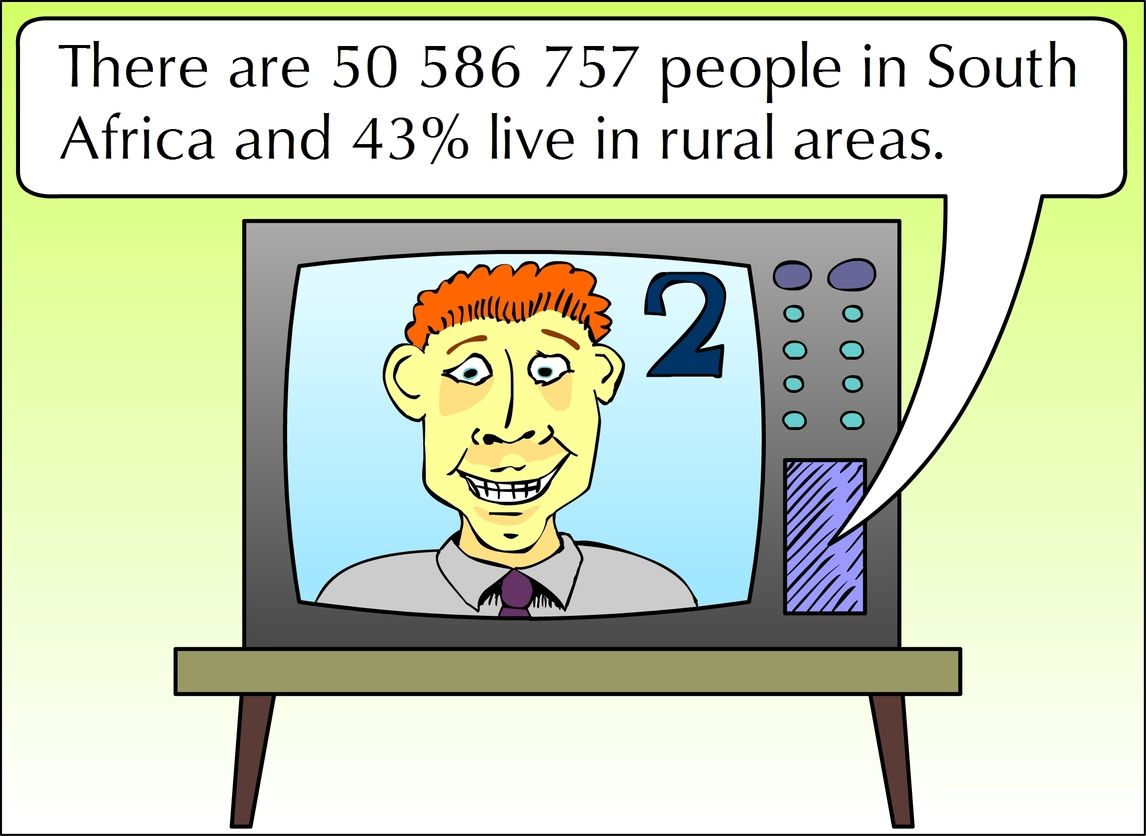How many people live in rural areas?

2.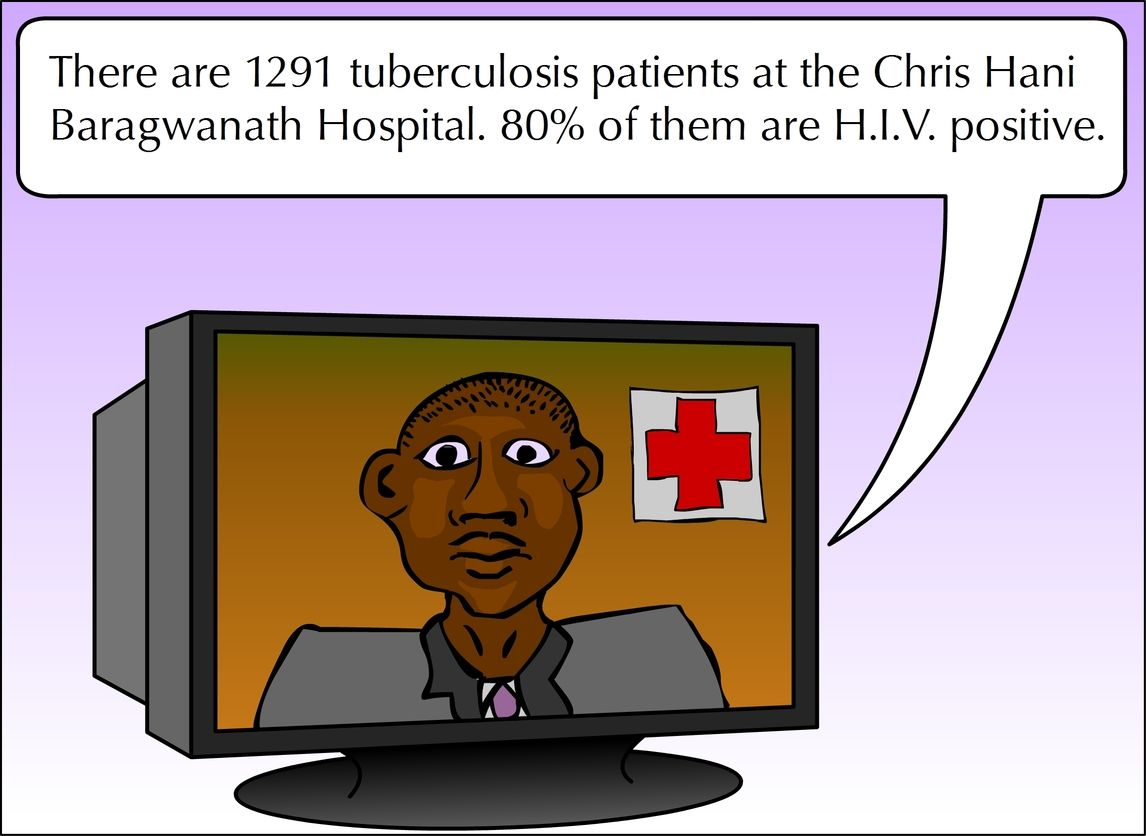How many T.B. patients are H.I.V. positive?

3.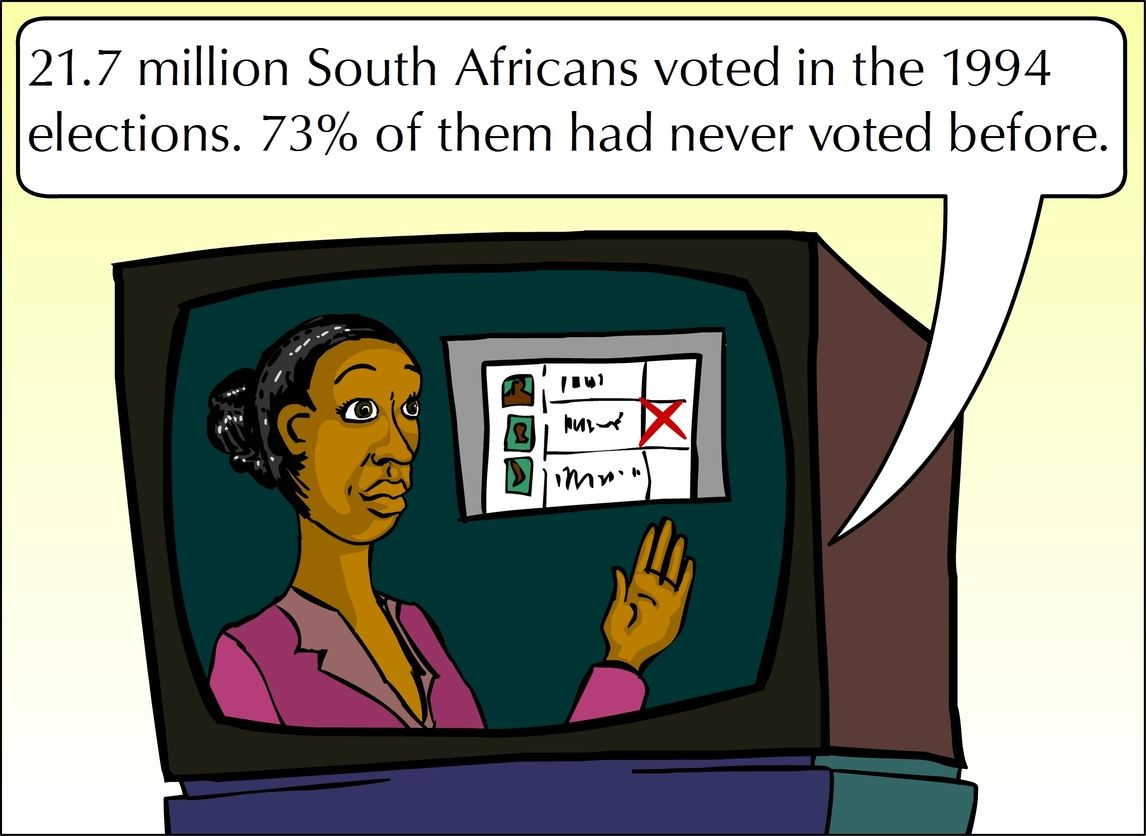How many people had never voted before the 1994 election?

1. $$\text{43}\% = \text{43} \div \text{100}$$ $$\frac{\text{43}}{\text{100}} \times \text{50 586 757} = \text{21 752 305}$$ people live in rural areas. With a calculator: To find $$\text{43}\%$$ of $$\text{50 586 757}$$ key in: $$\text{43} \div \text{100} \times \text{50 586 757} =$$ OR $$\text{43}\% \times \text{50 586 757} =$$
2. $$\text{80}\% = \text{80} \div \text{100}$$ $$\frac{\text{80}}{\text{100}} \times \text{1 291} = \text{1 032}$$ patients
3. $$\text{73}\% = \text{73} \div \text{100}$$ $$\frac{\text{73}}{\text{100}} \times \text{21 700 000} = \text{15 841 000}$$ people had never voted before.

## Worked example 17: Working out one amount as a percentage of another amount

Top Teenage T-shirts printed $$\text{120}$$ T-shirts. They sold $$\text{72}$$ T-shirts immediately. What percentage of the T-shirts were sold?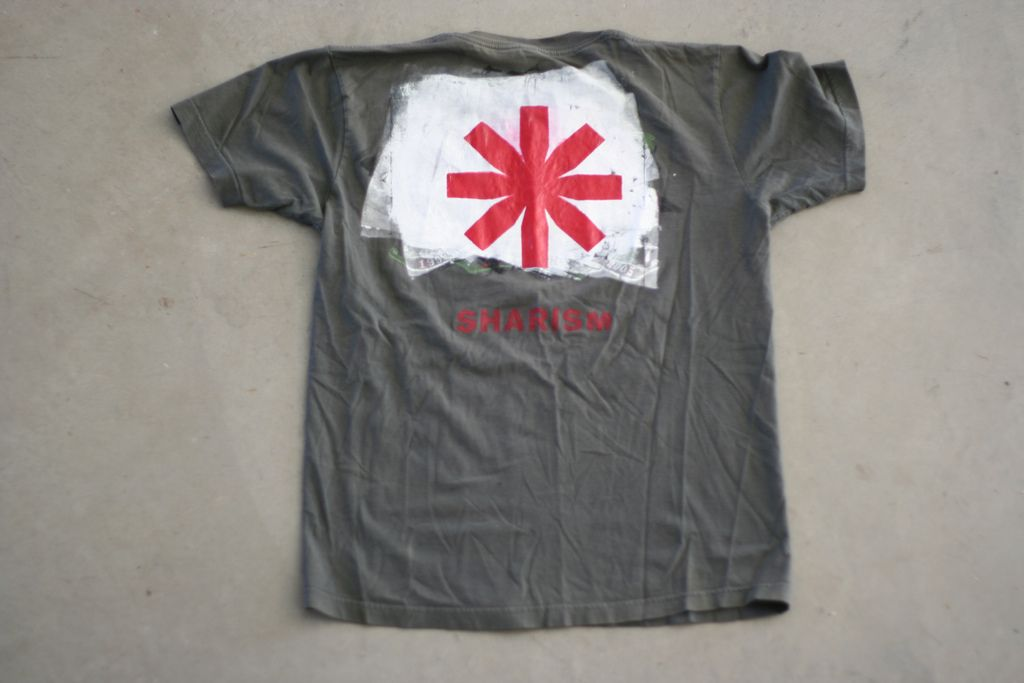$$\text{72}$$ of the $$\text{120}$$ T-shirts were sold

$$\text{72} \div \text{120} \times \text{100} = \text{60}\%$$. So $$\text{60}\%$$ of the T-shirts were sold.

## Calculating the percentages of amounts

Exercise 1.10

Calculate the following without a calculator:

$$\text{25}\%$$ of $$\text{R}\,\text{124,16}$$

$$\text{25}\% = \frac{\text{1}}{\text{4}}$$. $$\frac{\text{1}}{\text{4}} \text{ of } \text{R}\,\text{124,16} = \text{R}\,\text{124,16} \div \text{4} = \text{R}\,\text{31,04}$$

$$\text{50}\%$$ of $$\text{30}$$ $$\text{mm}$$

$$\text{50}\% = \frac{\text{1}}{\text{2}}$$. $$\frac{\text{1}}{\text{2}} \text{ of } \text{30}\text{ mm} = \text{30}\text{ mm} \div \text{2} = \text{15}\text{ mm}$$

$$\text{15}\%$$ of $$\text{R}\,\text{3 500}$$

$$\text{R}\,\text{525}$$

$$\text{12}\%$$ of $$\text{25}$$ litres

$$\text{3}$$ litres

$$\text{37,5}\%$$ of $$\text{22}$$ $$\text{kg}$$

$$\text{8,25}$$ $$\text{kg}$$

$$\text{75}\%$$ of $$\text{R}\,\text{16,92}$$

$$\text{R}\,\text{12,69}$$

$$\text{18}\%$$ of $$\text{105}$$ $$\text{m}$$

$$\text{18,9}$$ $$\text{m}$$

$$\text{79}\%$$ of $$\text{840}$$ $$\text{km}$$

$$\text{663,6}$$ $$\text{km}$$

Calculate what percentage the first amount is of the second amount (you may use your calculator):

$$\text{120}$$ of $$\text{480}$$

$$\text{25}\%$$

$$\text{23}$$ of $$\text{276}$$

$$\text{8,3}\%$$

$$\text{3 500}$$ $$\text{ml}$$ of $$\text{5}$$ litres

$$\text{70}\%$$

$$\text{750}$$ $$\text{g}$$ of $$\text{2}$$ $$\text{kg}$$

$$\text{37,5}\%$$

$$\text{4}$$ out of $$\text{5}$$ for a test

$$\text{90}\%$$

$$\text{2}$$ out of $$\text{14}$$ balls

$$\text{14,3}\%$$

### Percentage discounts and increases (EMG33)

Look at the following extracts from newspaper articles and adverts: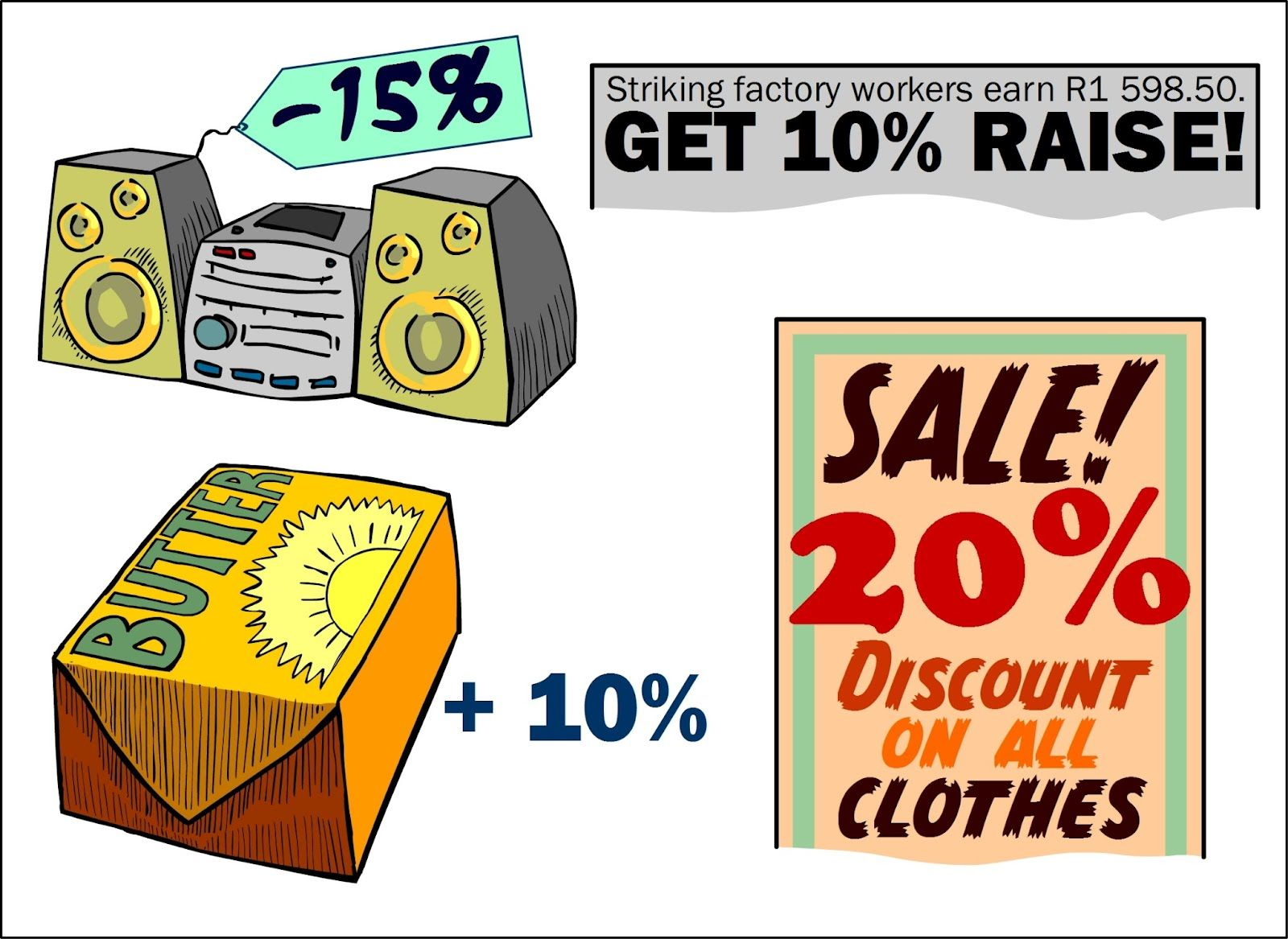Cost price
The amount that the dealer / trader / merchant pays for an article.
Marked price
This is the price of the article.
Selling price
This is the price after discount.
Profit
Sale price $$-$$ cost price.

## Discounts and increases

Exercise 1.11

The price of a tub of margarine is $$\text{R}\,\text{6,99}$$. If the price rises by $$\text{10}\%$$, how much will it cost?

New price is $$\text{R}\,\text{6,99}$$ + $$\text{10}\%$$ of $$\text{R}\,\text{6,99}$$= $$\text{R}\,\text{6,99}$$ + $$\text{70}$$ $$\text{c}$$ (rounded off) = $$\text{R}\,\text{7,69}$$ OR New price is ($$\text{100}$$ + $$\text{10}$$)\% of $$\text{R}\,\text{6,99}$$ = $$\text{110}\%$$ of $$\text{R}\,\text{6,99}=\frac{\text{110}}{\text{100}} \times \frac{\text{6,99}}{\text{1}}= \text{R}\,\text{7,69}$$ (rounded off)

Top Teenage T-shirts have a $$\text{20}\%$$ discount on all T-shirts. If one of their T-shirts originally cost $$\text{R}\,\text{189,90}$$, what will you pay for it now?

You only pay $$\text{80}\%$$ ($$\text{100}\%$$ $$-$$ $$\text{20}\%$$ discount). Thus: $$\frac{\text{80}}{\text{100}} \times \text{189,901} = \text{R}\,\text{151,92}$$ OR $$\text{20}\%$$ of $$\text{R}\,\text{189,90} = \frac{\text{20}}{\text{100}} \times \text{189,901}$$. The discount is thus $$\text{R}\,\text{37,98}$$. You pay $$\text{R}\,\text{189,90} - \text{R}\,\text{37,98} = \text{R}\,\text{151,92}$$.

Look at the pictures below. What is the value of each of the following items, in rands?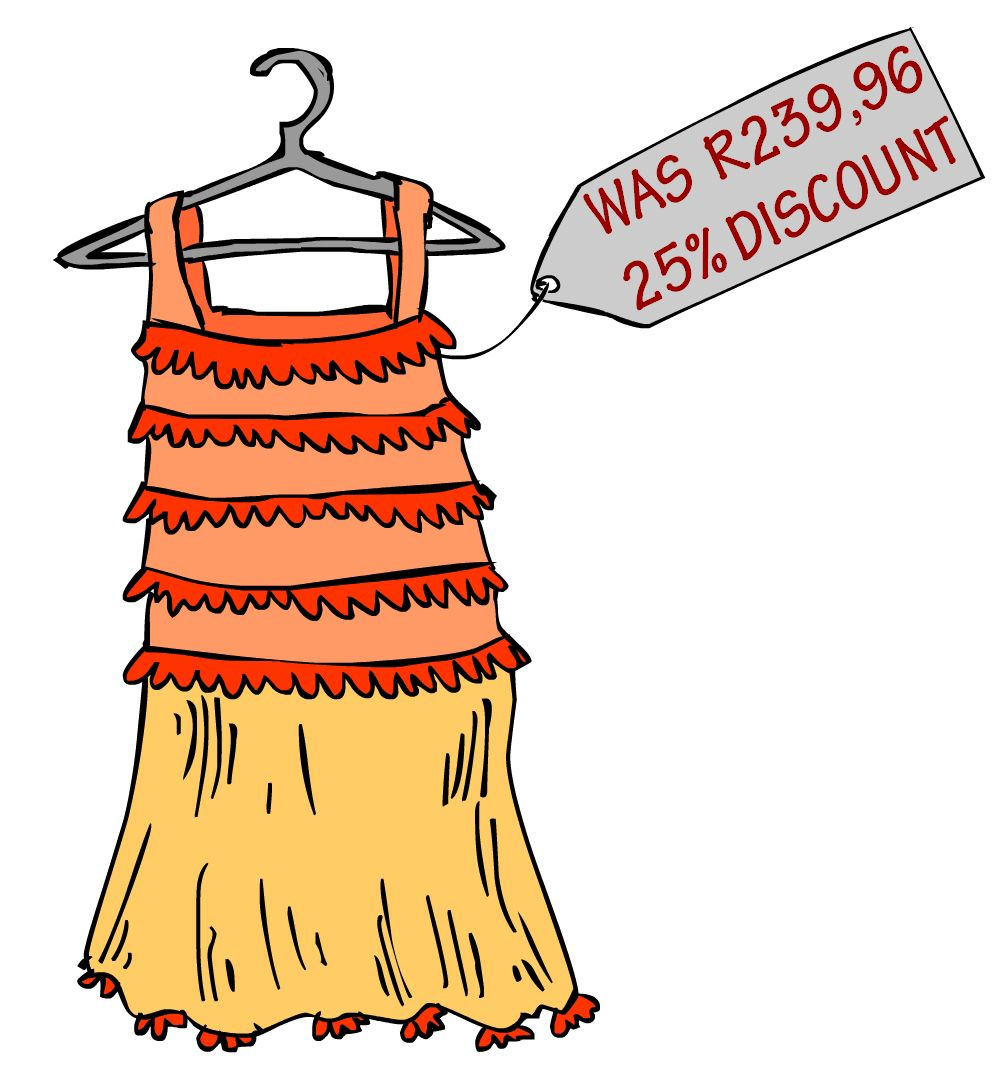$$\text{R}\,\text{239,96} - \text{R}\,\text{59,75} = \text{R}\,\text{180,21}$$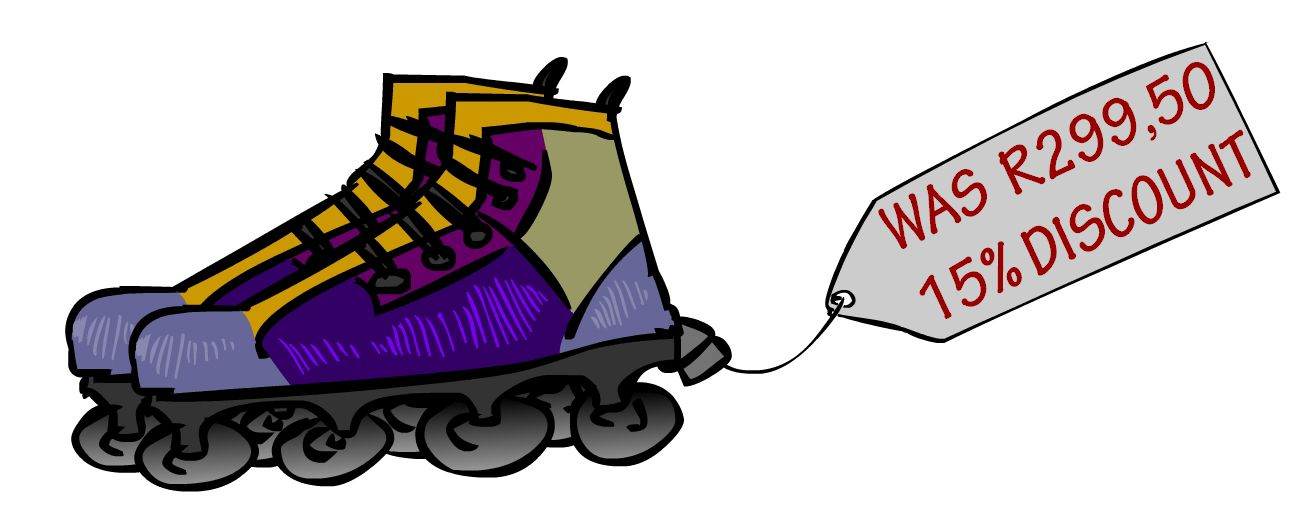$$\text{R}\,\text{299,50} - \text{R}\,\text{44,925} = \text{R}\,\text{1 254,58}$$$$\text{R}\,\text{9 875} + \text{R}\,\text{790} = \text{R}\,\text{10 665}$$$$\text{R}\,\text{15 995} + \text{R}\,\text{799,75}= \text{R}\,\text{16 794,75}$$

Calculate the percentage discount on each of these items:$$\frac{\text{R}\,\text{1 360}}{\text{R}\,\text{1 523}} = \text{89}\%$$. So discount is $$\text{100}\% - \text{89}\% = \text{11}\%$$$$\frac{\text{R}\,\text{527,40}}{\text{R}\,\text{586}} = \text{90}\%$$. So discount is $$\text{100}\% - \text{90}\% = \text{10}\%$$